>  Test: BITSAT Mock Test- 10

# Test: BITSAT Mock Test- 10

Test Description

## 150 Questions MCQ Test BITSAT Mock Tests Series & Past Year Papers | Test: BITSAT Mock Test- 10

 1 Crore+ students have signed up on EduRev. Have you?
Test: BITSAT Mock Test- 10 - Question 1

### Electric power is transferred at high potential because

Detailed Solution for Test: BITSAT Mock Test- 10 - Question 1 Electric power is transferred at high potential because to minimize power losses as:According to Joule’s first law electrical current passing through a conductor generates heat with power proportional to conductor’s electrical resistance and square of the current:P ~ I^2 x RSo in order to minimize this power, which would otherwise be lost to warming up the atmosphere, it is needed to reduce the current. In order to still transmit the same power to the destination with lower current we need to increase the voltage as evident from the electrical power equation:P = V x IThat is if it is needed to keep the power (P) the same, but lower the current (I) we have to proportionally increase voltage (V).
Test: BITSAT Mock Test- 10 - Question 2

### The ratio of the longest to shortest wavelengths in Brackett series of hydrogen spectra is

Detailed Solution for Test: BITSAT Mock Test- 10 - Question 2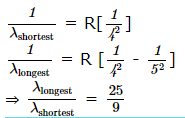Test: BITSAT Mock Test- 10 - Question 3

### A double charged lithium atom is equivalent to hydrogen whose atomic number is 3. The wavelength of required radiation for emitting electron from first to third Bohr orbit in Li⁺⁺ will be (Ionisation energy of hydrogen atom is 13.6 eV)

Test: BITSAT Mock Test- 10 - Question 4
An ammeter reads upto 1 ampere. Its internal resistance is 0.81 ohm. To increase the range to 10 A the value of the required shunt is
Test: BITSAT Mock Test- 10 - Question 5
A 220 V, 1000 W bulb is connected across a 110 V mains supply. The power consumed will be
Test: BITSAT Mock Test- 10 - Question 6

Energy of photon whose frequency is 1012MHz, will be

Test: BITSAT Mock Test- 10 - Question 7

A photo cell is illuminated by a source of light which is placed at a distance d from cell. If distance becomes d/2, then number of electrons emitted per second will

Detailed Solution for Test: BITSAT Mock Test- 10 - Question 7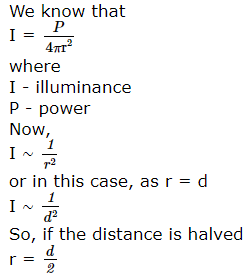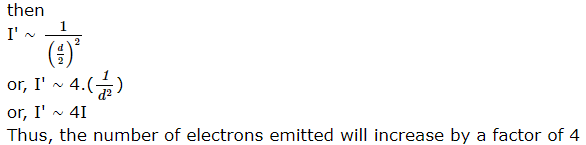Test: BITSAT Mock Test- 10 - Question 8
When the no. of turns in a coil is triplled, without any change in the length of coil, its self-inductance becomes
Test: BITSAT Mock Test- 10 - Question 9
Three point charges -q, Q and -q are placed at equal distances on a straight line. If total potential energy of the system is zero, then the ratio of Q/q is
Test: BITSAT Mock Test- 10 - Question 10

Three capacitors of capacitances 1 μF, 2 μF and 4 μF are connected first in a series combination, and then in a parallel combination. The ratio of their equivalent capacitances will be

Detailed Solution for Test: BITSAT Mock Test- 10 - Question 10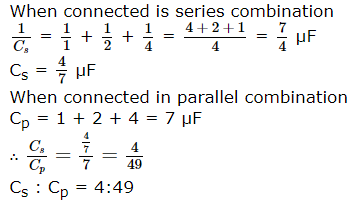Test: BITSAT Mock Test- 10 - Question 11

On a rough horizontal surface, a body of mass 2 kg is given a velocity of 10 m/s. If the coefficient of friction is 0.2 and g=10 m/s2, the body will stop after covering a distance of

Detailed Solution for Test: BITSAT Mock Test- 10 - Question 11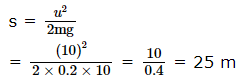Test: BITSAT Mock Test- 10 - Question 12
Venus looks brighter than other planets because
Test: BITSAT Mock Test- 10 - Question 13

A black body radiates 20 W at temperature 227oC. If temperature of the black body is changed to 727oC then its radiating power will be

Test: BITSAT Mock Test- 10 - Question 14
For one gram mol of gas, the value of R in the equation PV= RT is nearly
Test: BITSAT Mock Test- 10 - Question 15
A cyclotron can not accelerate
Test: BITSAT Mock Test- 10 - Question 16

Work done in turning a magnet of magnetic moment M by an angle of 90o from the magnetic meridian is n times the corresponding work done to turn through an angle of 60o, where n is

Detailed Solution for Test: BITSAT Mock Test- 10 - Question 16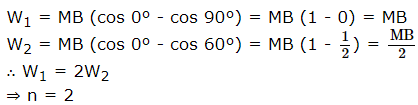Test: BITSAT Mock Test- 10 - Question 17
Oil spreads over the surface of water, whereas water does not spread over the surface of oil, because
Test: BITSAT Mock Test- 10 - Question 18
The surface tension of a liquid decreases with rise in
Test: BITSAT Mock Test- 10 - Question 19
The breaking stress of a wire depends upon
Test: BITSAT Mock Test- 10 - Question 20
Four persons K,L,M and N are initially at the corners of a square of side of length d. If every person starts moving. such that K is always headed towards L,L towards M.M is headed directly towards N and N towards K, then the four persons will meet after
Test: BITSAT Mock Test- 10 - Question 21

A body is projected at such an angle that the horizontal range is three times the greatest height. The angle of projection is

Detailed Solution for Test: BITSAT Mock Test- 10 - Question 21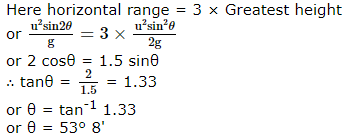Test: BITSAT Mock Test- 10 - Question 22

The parametric equations of a curve traced by a projectile on a certain planet with no surrounding are given by :y = (8t-5t2) metre and x = 6t metre, where t is in seconds. The velocity with which the projectile is projected, is

Test: BITSAT Mock Test- 10 - Question 23

A man is standing on a weighing machine placed in a lift. When stationary his weight is recorded as 40 kg. If the lift is accelerated upwards with an acceleration of 2 m/s2, then the weight recorded in the machine will be (g=10m/s2)

Test: BITSAT Mock Test- 10 - Question 24
To increase both the resolving power and magnifying power of a telescope
Test: BITSAT Mock Test- 10 - Question 25
A particle is viberating in a simple harmonic motion with an amplitude of 4 cm. At what displacement from the equilibrium position, is its energy half potential and kinetic ?
Test: BITSAT Mock Test- 10 - Question 26
Two masses m₁ and m₂ are suspended together by a massless spring of spring cosntant k. When the masses are in equilibrium, m₁ is removed without disturbing the system, then the angular velocity of oscillation of m₂ is
Test: BITSAT Mock Test- 10 - Question 27

In a spectrometer experiment three prisms A, B, C with same angle of prism but of different materials of refractive indices μ A = 1.33 , μ B = 1.55 and μ C = 1.44 are used. The corresponding angles of minimum deviation D A , D B , D C measured will be such that

Test: BITSAT Mock Test- 10 - Question 28
A circular disc is to be made by using iron aluminium, so that it acquires maximum moment of inertia about its geometrical axis. It can be obtained with
Test: BITSAT Mock Test- 10 - Question 29

The linear acceleration of centre of gravity of a ball which rolls down without slipping over an inclined plane with an angle of inclination of 30o will be

Test: BITSAT Mock Test- 10 - Question 30

A meter stick is held vertically with one end on the floor and is then allowed to fall. If the end touching the floor is not allowed to slip, the other end will hit the ground with a velocity of (g = 9.8 m/s2)

Detailed Solution for Test: BITSAT Mock Test- 10 - Question 30

C. G. of the metre scale is at its midpoint P.E. of stick is converted into rotational K. E. Thus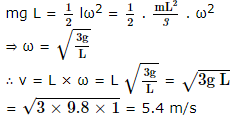Test: BITSAT Mock Test- 10 - Question 31
A liquid boils when its vapour pressure equals
Test: BITSAT Mock Test- 10 - Question 32
When a copper voltmeter is connected with a battery of e.m.f. 12 V, 2 g of copper is deposited in 30 min. If the same voltameter is connected across a 6 V bettery, then mass of copper deposited in 45 min would be
Test: BITSAT Mock Test- 10 - Question 33
The internal energy change in a system that has absorbed 2 KCals of heat and done 500 J of work is:
Test: BITSAT Mock Test- 10 - Question 34

In planetary motion the areal velocity of position vector of a planet depends on angular velocity ′ ω ′ and the distance of the planet from sun (r). If so the correct relation for areal velocity is

Test: BITSAT Mock Test- 10 - Question 35
Which of the following phenomena establish the wave nature of light?
Test: BITSAT Mock Test- 10 - Question 36
The ray of light
Test: BITSAT Mock Test- 10 - Question 37

A stretched string of length l, fixed at both ends can sustain stationary waves of wavelength λ, given by

Test: BITSAT Mock Test- 10 - Question 38

Two wires are fixed in a sonometer. Their tensions are in the ratio 8:1. The lengths are in the ratio 36 : 35. The diameters are in the ratio 4:1. Densities of the mateirals are in the ratio 1:2. If the higher frequency in the setting is 360 Hz. the beat frequency when the two wires are sounded together is

Detailed Solution for Test: BITSAT Mock Test- 10 - Question 38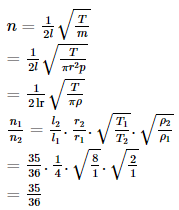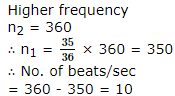Test: BITSAT Mock Test- 10 - Question 39

A body of mass 6 kg is under a force which causes displacement in it given by S = t 2/4 metres where t is time. The work done by the force in 2 seconds is

Detailed Solution for Test: BITSAT Mock Test- 10 - Question 39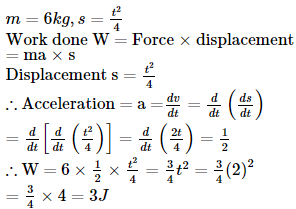Test: BITSAT Mock Test- 10 - Question 40
A machine, which is 75% efficient, uses 12 J of energy in lifting up a 1 kg mass through a certain distance. The mass is then allowed to fall through that distance. The velocity at the end of its fall is (in m/s)
Test: BITSAT Mock Test- 10 - Question 41
An organic compound A reacts with methyl magnesium iodide to form an addition product which on hydrolysis forms the compound B. Compound B gives blue colour salt in Victor Meyer's test. The compounds A and B are respectively
Test: BITSAT Mock Test- 10 - Question 42
Aldehydes and ketones will not form crystalline derivatives with
Test: BITSAT Mock Test- 10 - Question 43
The first use of quantum theory to explain the structure of atom, was given by
Test: BITSAT Mock Test- 10 - Question 44
The equation ∆x.∆p ≥ h/4π shows
Test: BITSAT Mock Test- 10 - Question 45

One mole of CO₂ contains

Test: BITSAT Mock Test- 10 - Question 46
The ratio of the molar amounts of H₂S needed to precipitate the metal ions from 20 mL each of 1M Cd(NO₃)₂ and 0.5 M CuSO₄ is
Detailed Solution for Test: BITSAT Mock Test- 10 - Question 46 As Cd2+ and Cu2+, both are dipositive ions but Cu2+ has one half of the concentration compared to that of the Cd2+. Thus, the molar amount of the H2S used to precipitate the metals ions Cd2+ and Cu2+ is 2 : 1.
Test: BITSAT Mock Test- 10 - Question 47
which of the following hybridisation occurs in the formation of methane molecule?
Test: BITSAT Mock Test- 10 - Question 48
Which of the following is an electrophile?
Test: BITSAT Mock Test- 10 - Question 49
The segment of DNA which acts as the instructional manual for the synthesis of the protein is
Test: BITSAT Mock Test- 10 - Question 50
The sugar that is characteristic of milk is
Test: BITSAT Mock Test- 10 - Question 51
An organic acid having molecular formula C₂H₄O₂ is
Test: BITSAT Mock Test- 10 - Question 52
Which of the following does not conduct electricity?
Test: BITSAT Mock Test- 10 - Question 53

What is the entropy change (in JK⁻1 mol⁻1) When one mol of ice is converted into water at 0oC. The enthalpy change for the conversion of ice to liquid water is 6.0 KJ mol⁻1 at )0oC.

Test: BITSAT Mock Test- 10 - Question 54
1 mole of hydrogen and 2 moles of iodine are taken initially in a 2 litre vessel. The number of moles of hydrogen at equilibrium is 0.2. Then the number of moles of iodine and hydrogen iodide at equilibrium are
Test: BITSAT Mock Test- 10 - Question 55
The activation energy of a reaction can be determined by
Test: BITSAT Mock Test- 10 - Question 56
As per the modern periodic law the physical and chemical properties of elements are periodic function of their
Detailed Solution for Test: BITSAT Mock Test- 10 - Question 56 Modern periodic law states " If the elements are arranged in order of their increasing atomic numbers, the elements with similar properties are repeated after certain regular intervals.
Test: BITSAT Mock Test- 10 - Question 57

The heat of formation of the compound in the following reaction is H₂(g) + Cl₂(g) → 2HCl(g) + 44 kcal

Test: BITSAT Mock Test- 10 - Question 58
Which of the following could act as a propellant for rockets?
Test: BITSAT Mock Test- 10 - Question 59
The oxidation number of cobalt in K[Co(CN)₄] is
Test: BITSAT Mock Test- 10 - Question 60
Which of the following is an amphoteric oxide?
Test: BITSAT Mock Test- 10 - Question 61
Which lanthanide compound, after dissolving in selenium oxychloride, is used as a powerful liquid lasers?
Test: BITSAT Mock Test- 10 - Question 62
A certain quantity of elecricity is passed through an aqueous solutions of AgNO3 and CuSO4 connected in series. The amount of silver (at wt. = 108) deposited at the cathode is 1.08 gram. The amount of copper (at wt. = 63.54) deposited is
Test: BITSAT Mock Test- 10 - Question 63
In which of the following molecules hydrogen is more acidic?
Test: BITSAT Mock Test- 10 - Question 64
Which of these does not follow Anti-Markownikoff's rule?
Test: BITSAT Mock Test- 10 - Question 65
What is formed when calcium carbide reacts with heavy water?
Test: BITSAT Mock Test- 10 - Question 66
Aqueous solution of sodium acetate is
Test: BITSAT Mock Test- 10 - Question 67
CH₃CH₂CH₂OH and CH₃CH₂OCH₃ are
Test: BITSAT Mock Test- 10 - Question 68
Formaldehyde gives an additive product with methyl magnesium iodide which on aqueous hydrolysis gives
Test: BITSAT Mock Test- 10 - Question 69
Which of the following has the highest calorific value?
Test: BITSAT Mock Test- 10 - Question 70
If HNO₃ changes into N₂O, the oxidation number is changed by
Test: BITSAT Mock Test- 10 - Question 71
Laughing gas is
Test: BITSAT Mock Test- 10 - Question 72
Identify the statement that is not correct as far as structure of diborane is concerned
Test: BITSAT Mock Test- 10 - Question 73
H₂S gas when passed through a solution of cations containing HCl precipitates the cations of second Group of qualitative analysis, but not those belonging to fourth Group. It is because
Test: BITSAT Mock Test- 10 - Question 74
Buna-N is prepared by the polymerisation of
Test: BITSAT Mock Test- 10 - Question 75
Glauber's salt is
Test: BITSAT Mock Test- 10 - Question 76
Identify the correct statement.
Test: BITSAT Mock Test- 10 - Question 77

Vapour pressure of CCl₄ at 25oC is 143 mm Hg. 0.5 gm of a non-volatile solute (mol.wt.65) is dissolved in 100 ml of CCl₄. Find the vapour pressure of the solution . (Density of CCl₄=1.58 g/cm3).

Test: BITSAT Mock Test- 10 - Question 78

50 ml of a gas A diffuse through a membrane in the same time as for the diffusion of 40 ml of a gas B under identical pressure temperature conditions. If the molecular weight of A = 4, that of B would be

Test: BITSAT Mock Test- 10 - Question 79

When 10 g of 90% pure limestone is heated completely, the volume (in litres) of carbon dioxide liberated at STP is

Detailed Solution for Test: BITSAT Mock Test- 10 - Question 79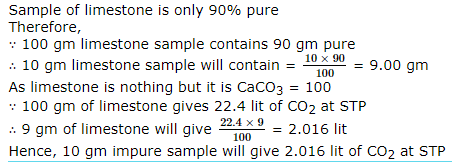Test: BITSAT Mock Test- 10 - Question 80
Difference between colloids and cystalloids is of
Test: BITSAT Mock Test- 10 - Question 81

The are bounded by the curve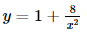with x-axis and the ordinates at x = 2 and x = 4 is

Test: BITSAT Mock Test- 10 - Question 82
If the coefficient of x7 and x8 in [2+(x/3)]n are equal, then n is
Test: BITSAT Mock Test- 10 - Question 83

The centre and radius of the circle with the segment of the line x+y=1 cut of by the coordinate axes as diameter are

Test: BITSAT Mock Test- 10 - Question 84
If two distinct chords, drawn from the point (p,q) on the circle x2 + y2 - px - qy = 0 (where $pq\ne 0$) are bisected by the x-axis, then
Test: BITSAT Mock Test- 10 - Question 85

The value of (1 - ω + ω2)(1 - ω2 + ω6), where ω,ω2 are cube roots of unity ,is

Test: BITSAT Mock Test- 10 - Question 86
The differential equation of family of lines which passes (1,-1) is
Test: BITSAT Mock Test- 10 - Question 87

The degree of the differential equation d2y/dx2+(dy/dx)3+6y=0 is

Test: BITSAT Mock Test- 10 - Question 88

The solution of the equation (dy/dx)=cotx coty is

Detailed Solution for Test: BITSAT Mock Test- 10 - Question 88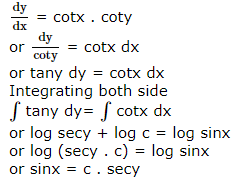Test: BITSAT Mock Test- 10 - Question 89

Differential of log(1-√x)sin⁻1(1-√x) w.r.t. 22(1-√x) at x=(1/4) is equal to

Test: BITSAT Mock Test- 10 - Question 90

Differential of x6 w.r. to x3 is equal to

Test: BITSAT Mock Test- 10 - Question 91

The sum of (12.2)/1! + (22.3)/2! + (32.4)/3! + (42.5)/4! + ..... will be

Test: BITSAT Mock Test- 10 - Question 92
From the top of a building of height h metres, the angle of depression of an object on the ground is α. The distance (in metres) of the object from the foot of the building is
Test: BITSAT Mock Test- 10 - Question 93

Equation of the tangent to the hyperbola 2x2 - 3y2 = 6 which is parallel to the line y = 3x + 4 is

Detailed Solution for Test: BITSAT Mock Test- 10 - Question 93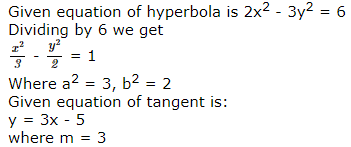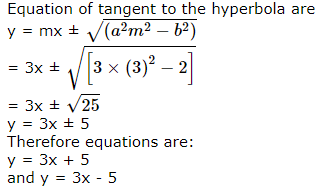Test: BITSAT Mock Test- 10 - Question 94

The area bounded by the curve
x = at2, y = 2at and the X-axis is 1 ≤ t ≤ 3 is

Test: BITSAT Mock Test- 10 - Question 95

The area bounded by the curve y2 = 9x and the lines x = 1, x = 4 and y = 0 in the first quadrant is

Test: BITSAT Mock Test- 10 - Question 96

tan⁻1(1/2)+tan⁻1(1/3)=

Test: BITSAT Mock Test- 10 - Question 97

If φ(x)=ax, then {φ(p)}3 is equal to

Test: BITSAT Mock Test- 10 - Question 98

If Sn = 13 + 23 + … + n3 and Tn = 1 + 2 + … + n then

Test: BITSAT Mock Test- 10 - Question 99
The equation
x + 2y + 3z = 1
x - y + 4z = 0
2x + y + 7z = 1 have
Test: BITSAT Mock Test- 10 - Question 100
If A and B are square matrices of order 3 such that ∣A∣ = -1, ∣B∣ = 3, then the determinant of 3 AB is equal to
Test: BITSAT Mock Test- 10 - Question 101

For all real x, the minimum value of [(1-x+x2)/(1+x+x2)] is

Test: BITSAT Mock Test- 10 - Question 102
Which of the following are correct for any two complex numbers z₁ and z₂ ?
Test: BITSAT Mock Test- 10 - Question 103

If bisectors of lines x2 - 2pxy - y2 = 0 is x2 - 2qxy - y2, then

Detailed Solution for Test: BITSAT Mock Test- 10 - Question 103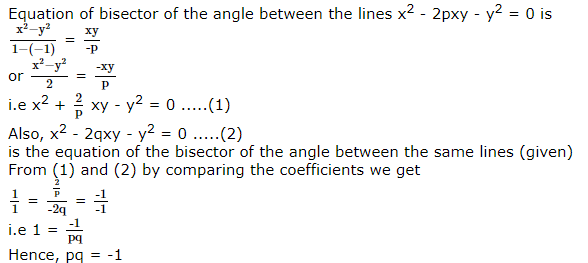Test: BITSAT Mock Test- 10 - Question 104

The vertex of the parabola x2 + 12 x − 9 y = 0 is

Test: BITSAT Mock Test- 10 - Question 105

The equation of the tangent to the parabola y2=16x which is ⊥ to the line y=3x+7 is

Test: BITSAT Mock Test- 10 - Question 106
If nP4=30.nC5, then n=
Test: BITSAT Mock Test- 10 - Question 107
The number of parallelograms that can be formed from a set of four parallel lines intersecting another set of there parallel lines is :
Test: BITSAT Mock Test- 10 - Question 108

A box contains 10 good articles and 6 with defects. One item is drawn at random. The probability that it is either good or has a defect is

Detailed Solution for Test: BITSAT Mock Test- 10 - Question 108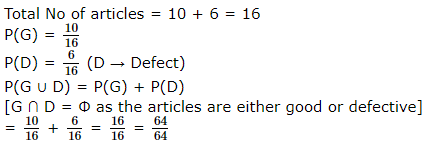Test: BITSAT Mock Test- 10 - Question 109
A sum of money is rounded off to the nearest rupee. The probability that round off error is at least ten paise is
Test: BITSAT Mock Test- 10 - Question 110

In a triangle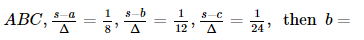Test: BITSAT Mock Test- 10 - Question 111

In a ΔABC,a(cos2B+cos2C)+cosA(ccosC+bcosB)= =

Test: BITSAT Mock Test- 10 - Question 112

If one root of the equation x2 = px + q is reciprocal of the other, then the correct relationshup is :

Test: BITSAT Mock Test- 10 - Question 113

The fourth, seventh and tenth terms of a G.P. are p, q and r respectively, then

Test: BITSAT Mock Test- 10 - Question 114

The fourth ,seventh and tenth of a G.P. are p,q,r respectively then

Test: BITSAT Mock Test- 10 - Question 115
The range of the function f (x) = cos [x], where (- π/2) < x < ( π/2), is
Test: BITSAT Mock Test- 10 - Question 116

If A = [a,b], B = [c,d], C = [d,e] then {(a,c), (a,d),(a,e),(b,c),(b,d),(b,e)} is equal to

Test: BITSAT Mock Test- 10 - Question 117
The equation of a line which is passing through (-3,2) and cuts intercepts on axes of opposite signs, is
Test: BITSAT Mock Test- 10 - Question 118

The point on the curve where the normal to the curve 9y2 = x3 makes equal intercepts with the axes is

Test: BITSAT Mock Test- 10 - Question 119

The direction ratios of the line which is perpendicular to the lines (x - 7)/2 = (y + 17)/-3 = (z - 6)/1 and (x + 5)/1 = (y + 3)/2 = (z - 4)/-2 are

Detailed Solution for Test: BITSAT Mock Test- 10 - Question 119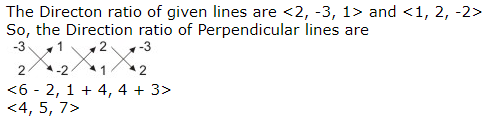Test: BITSAT Mock Test- 10 - Question 120

The projections of a line segment on the coordinate are 12, 4 and 3 respectively. The length of the line segment is

Detailed Solution for Test: BITSAT Mock Test- 10 - Question 120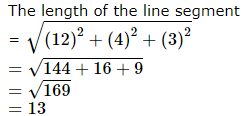Test: BITSAT Mock Test- 10 - Question 121

The smallest values of θ satisfying the equation √3 cot θ + tan θ = 4 is

Test: BITSAT Mock Test- 10 - Question 122

If √3 cos θ − sin θ = 1 then θ =

Test: BITSAT Mock Test- 10 - Question 123

If x=cos10o cos20o cos40o, x=

Test: BITSAT Mock Test- 10 - Question 124
If vectors (i+j+k),(5i+3j-3k) and (2i+5j+9k) are the position vectors of vertices of any triangle then its perimeter is